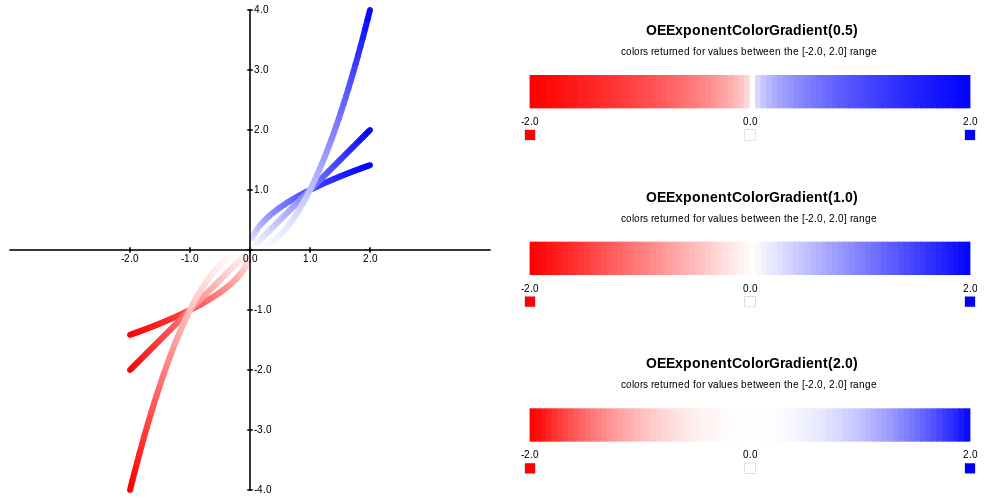class OEExponentColorGradient : public OEColorGradientBase


The OEExponentColorGradient class interpolates between colors that are defined by using the OEColorStop class. The OEExponentColorGradient.TransformValue method defines the transformation that is performed before returning an interpolated color associated with a floating point value. The Figure: Example of various exponent color gradients illustrates the difference between OEExponentColorGradient objects constructed with the same color stops but with different exponent values. An OEExponentColorGradient object constructed with 1.0 exponent value is equivalent to an OELinearColorGradient object constructed with the same color stops.Example of various exponent color gradients generated with (-2.0, OERed), (0.0, OEWhite) and (+2.0, OEBlue) color stops

The following methods are publicly inherited from OEColorGradientBase:

## Constructors¶

OEExponentColorGradient(double exponent)


Constructor that creates an “empty” color gradient with the given exponent value.

exponent

The given value is clamped into the range of [0.1, 10.0].

Note

An “empty” color gradient always returns the OEBlack color, when calling the OEColorGradientBase.GetColorAt, the OEColorGradientBase.GetMinColor or the OEColorGradientBase.GetMaxColor methods.

OEExponentColorGradient(double exponent, const OEColorStop &oneStop)


Creates an OEExponentColorGradient object with one color stop and an exponent value. An OEExponentColorGradient object that has only one color stop will always return that color when calling the OEColorGradientBase.GetColorAt method.

exponent

The given value is clamped into the range of [0.1, 10.0].

OEExponentColorGradient(double exponent, const OEColorStop &bgnStop,
const OEColorStop &endStop)


Creates an OEExponentColorGradient object with interpolation range between the two specified color stops using the given exponent value.

exponent

The given value is clamped into the range of [0.1, 10.0].

OEExponentColorGradient(const OEExponentColorGradient &rhs)


Copy constructors.

## operator=¶

OEExponentColorGradient &operator=(const OEExponentColorGradient &rhs)


Assignment operator.

## GetExponent¶

double GetExponent() const


Returns the exponent value used in the OEExponentColorGradient.TransformValue method.

## SetExponent¶

void SetExponent(double exponent)


Sets the exponent value used in the OEExponentColorGradient.TransformValue method.

exponent

The given value is clamped into the range of [0.1, 10.0].

## TransformValue¶

double TransformValue(double value) const


Performs a $$f(x) = -x^y$$ transformation when x is less than 0.0 and $$f(x) = x^y$$ transformation when x is greater than 0.0, where the $$y$$ is the exponent returned by OEExponentColorGradient.GetExponent method. This method is called by OEColorGradientBase.GetColorAt method to transform a value before performing color interpolation.